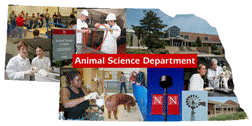## Animal Science Department## Faculty Papers and Publications in Animal Science

#### Date of this Version

7-8-2003

Published in J. Anim. Sci. 2003. 81:1926–1932.

#### Abstract

Statistical models (16) were compared for birth weight of 24,741 Columbia lambs recorded at the U.S. Sheep Experiment Station (USSES; 1950 to 1998). The goal was to estimate fractions of variance due to cytoplasmic line (c2; n = 590) and sire × cytoplasmic line interaction (sc2; n = 12,831) with other nuclear and environmental effects included in the model. The basic model included direct genetic (fractional variance, a2; n = 26,623), maternal genetic (m2, with direct-maternal correlation ram), and maternal permanent environmental (p2m ; n = 6,385) effects. The model with sc2 was significantly (P < 0.05) better than the basic model with c2, but with other random effects added, sc2 became zero. Significant (P < 0.05) variance components were associated with random dam × year (dy2) and dam × number born (dn2) interaction effects. Estimates with all effects in the model were: a2, 0.26; m2, 0.24; ram, −0.05; p2m , 0.02; c2, 0.00; dn2, 0.04; dy2, 0.08; sd2, 0.04; sc2, 0.00. Estimates for a2, m2, and ram were the same for all models. Estimate of p2m changed when other effects were added to the model. The largest estimates for nongenetic effects were: p2m , 0.06; c2, 0.00; dy2, 0.14; sd2, 0.13; and sc2, 0.04. Parameter estimates were similar regardless of whether fixed genetic groups (n = 84) were in the model. For weaning weight (120 d, n = 23,903), estimates of variances of effects added to the basic model were all near zero (a2, 0.14; m2, 0.09; ram, 0.23; p2m , 0.04). For fleece weight (FW, n = 29,024) and number born (NB, n = 29,688), animal permanent environmental effects were added to the model (p2a; n = 7,741 and 7,840) and ram was dropped. For these traits, effects not in the basic model had small variances. For FW, estimates with the full model were: a2, 0.52; m2, 0.01; p2m , 0.00; p2a, 0.06; c2, 0.00; dy2, 0.00; sd2, 0.02; and sc2, 0.06, and for NB they were: a2, 0.08; m2, 0.00; p2m , 0.00; p2a, 0.02; c2, 0.00; dy2, 0.00; sd2, 0.00; and sc2, 0.01. Cytoplasmic effects were not important. Unusual random effects in the model did not change estimates for basic parameters. Although some effects were significant, especially for BW, effects on genetic evaluations are likely to be small.

COinS